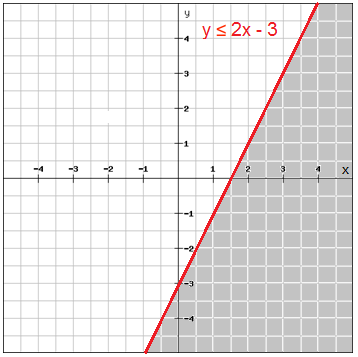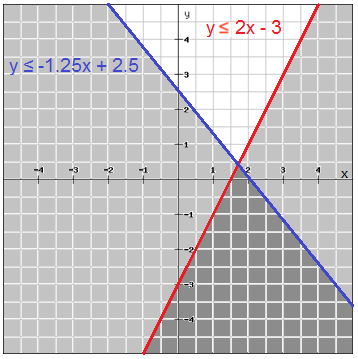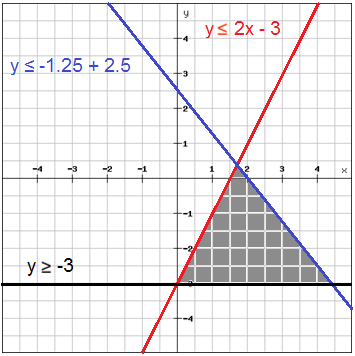# Systems of linear inequalities

A system of linear inequalities in two variables consists of at least two linear inequalities in the same variables. The solution of a linear inequality is the ordered pair that is a solution to all inequalities in the system and the graph of the linear inequality is the graph of all solutions of the system.

Example

Graph the system of inequalities

$\\\left\{\begin{matrix} y\leq 2x-3\: \: \: \: \: \: \: \: \\ y\geq -3\: \: \: \: \: \: \: \: \: \: \: \: \: \: \: \\ y\leq -1.25x+2.5 \end{matrix}\right.\\$

Graph one line at the time in the same coordinate plane and shade the half-plane that satisfies the inequality.The solution region which is the intersection of the half-planes is shown in a darker shadeUsually only the solution region is shaded which makes it easier to see which region is the solution region## Video lesson

Graph the system of inequalities

$\\\left\{\begin{matrix} x > 3 \\ y \leq -x + 2 \end{matrix}\right.\\​$